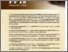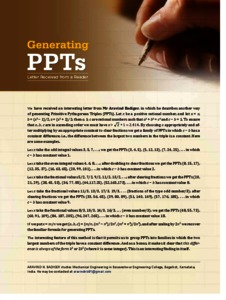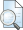Badiger, Arvind N. (2012) Generating PPTs-letter received from the reader. At Right Angles, 1 (2). p. 1. ISSN 2582-1873Preview
Text - Published Version

## Abstract

Describes another way of generating Primitive Pythagorean Triples (PPTs). Let x be a positive rational number, and let a = x, b = (x 2 − 1)/2, c = (x 2 + 1)/2; then a, b, c are rational numbers such that a 2 + b 2 = c 2 and c − b = 1. To ensure that a, b, c are in ascending order we must have x > 2 + 1 . 2 . 414 . By choosing x appropriately and af- ter multiplying by an appropriate constant to clear fractions we get a family of PPTs in which c − b has a constant difference; i.e., the difference between the largest two numbers in the triple is a constant. Here are some examples.

Item Type: Articles in APF MagazinesView Item